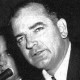## The Continuum Hypothesis

The Continuum Hypothesis is very interesting. According to mathworld, It is a proposal (originally by George Cantor) that there is no infinite set with a cardinal number between that of the infinite set of integers and the larger infinite set of real numbers. It is easy to show that the second infinite set is larger than the first – 1st year students are shown this (think Cantor’s diagonal argument which would be familiar to most tertiary maths students who have studied topology or analysis).

Is the Continuum Hypothesis true? To quote from mathworld,

Gödel showed that no contradiction would arise if the continuum hypothesis were added to conventional Zermelo-Fraenkel set theory. However, using a technique called forcing, Paul Cohen (1963, 1964) proved that no contradiction would arise if the negation of the continuum hypothesis was added to set theory. Godel’s and Cohen’s results established that the validity of the continuum hypothesis depends on the version of set theory being used, and is therefore undecidable (assuming the Zermelo-Fraenkel axioms together with the axiom of choice).

1.GMB says: